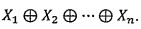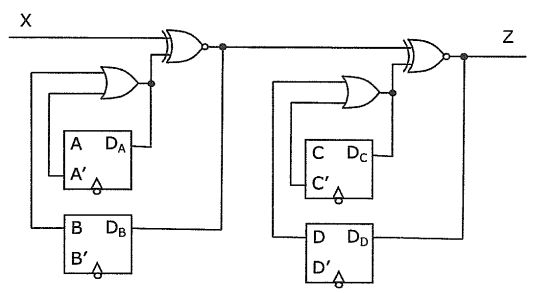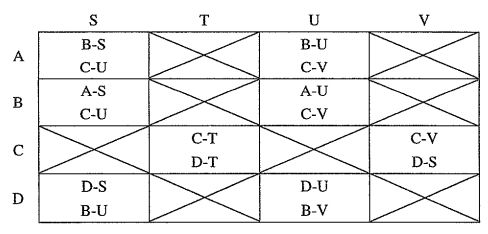milk26364325>試卷(2021/09/15)

# 110 年 - 110 國立臺灣大學_碩士班招生考試_電子工程研究所丁組:邏輯設計#101259

【非選題】
1.
Problem 1: (20 points)

【題組】(a) (4%) Show that the set ( AND, OR, NOT) of logic operations is functionally complete, that is, any Boolean function can be represented with this set of logic operations.

【非選題】
2.【題組】(b) (4%) Is the set consisting of only majority gate MAJ functionally complete? Justify your answer. (Note that the majority gate MAJ(a,b,c) with three inputs a, b, c equals 1 if and only if at least two of the inputs are 1.)

【非選題】
3.【題組】(c) (4%) Is the set consisting of only minority gate MIN functionally complete? Justify your answer. (Note that the minority gate MIN(a,b,c) with three inputs a, b, c equals 1 if and only if at most one of the inputs is 1.)

【非選題】
4.【題組】(d) (4%) Can any Boolean function be represented in the exclusive-sun-of products (ESOP) expression, which is the AND-XOR two-level expression (with first level AND and second level XOR)? Justify your answer.

【非選題】
5.【題組】(e)(4%) Can any Boolean function be represented in the exclusive-product-of-suns (EPOS) expression, which is the XOR-AND two-level expression (with first level XOR and second level AND)? Justify your answer.

【非選題】
6.

Problem 2: (20 points) Consider the expression【題組】(a) (10%) Prove by induction that it represents an odd-parity function, that is, it evaluates to 1 if and only if the assignment to variables X1,...,Xn contains an odd number of 1's. (For example, the assignment (X1, X2, X3, X4)= (1,0,0,0) contains one 1.)

【非選題】
7.【題組】(b) (5%) How many product terms are there for its minimum sum-of-products (SOP) expression?

【非選題】
8.【題組】(c) (5%) How many sum terms are there for its minimum product-of-sums (POS) expression?

【非選題】
9.

Problem 3: (20 points) Consider the following sequential circuit.Assume that the four D-flip-flops are triggered by the same clock signal, the flip-flop propagation delay is 1 ns, the flip-flop setup cime is 2 ns, the input signal X has delay 2 ns, the OR gate has delay 3 ns, and the XOR gate has delay 4 ns.

【題組】(a) (5%) Determine the minimum clock cycle that the circuit can operate.

【非選題】
10.【題組】(b) (5%) Determine the constraint on the flip-flop hold time for the circuit to operate correctly.

【非選題】
11.【題組】(c) (5%) Assume the combinational part of the circuit is to be re-implemented by a single read-only memory (ROM). Determine the size of the ROM in terms of 1) the number of words and 2) the bit-width of each word.

【非選題】
12.【題組】(d) (5%) Assume the circuit is initially in state (A,B,C,D) = (0,0,0,0). Determine the corresponding state-transition sequence and output sequence with respect to the input sequence 0, 1, 1.

【非選題】
13.
Problem 4: (20 points)
Design a Moore sequential circuit with input X and output Z that meets the following requirement.
Z= 1, ifthere are at least two I's appearing in the last three inputs, and Z= 0, otherwise.
Example
x=001101100101000...
Z=000111110001000...

【題組】(a) (10%) Draw the corresponding state graph and identify all equivalent states, if there is any, for state minimization.

【非選題】
14.【題組】(b) (10%) Implement the circuit with three D flip-lops and one majority gate MAJ.

【非選題】
15.
Problem 5: (20 points)
Given two Mcaly sequential circuits, let M1 have four states A, B, C, D and M2 have four states S, T, U, V. Assume the implication table (or pair chart) for the state pairs between M1 and M2 is as follows (where an entry with a cross sign indicates the output difference for the corresponding state pair between M1 and M2, and the expression "Y-Z* in a (W.X)-entry indicates that the next-state pair (Y, 2) must be equivalent in order for the corresponding current-state pair (W,X) to be equivalent).【題組】(a)(5%) Find all possible initial state pairs between M1 and M2 that make the two circuits behave the same.

【非選題】
16.【題組】(b) (5%) Let M1 starts from state C and M2 starts from state T. What is the minimum length of an input sequence that makes M1 and M2 produce different outputs? Justify your answer.

【非選題】
17.【題組】(c) (5%) Are there equivalent states in M1? If yes, which states are equivalent?

【非選題】
18.【題組】(d) (5%) Assume S is the initial state of M2. Identify all the states in M2 that can be reached from S. (We say that state A can reach state B if there exists a sequence of state transitions from A to B.)

### 懸賞詳解

#### 國二自然上第二次

30.如附圖繩波向右傳播，則繩子上的質點來回振動 一次，其路徑可能為何？ (A) A→B→D→E (B) A→F→C→E (C) B→F→G→F (D) B→F→G→F →B ...